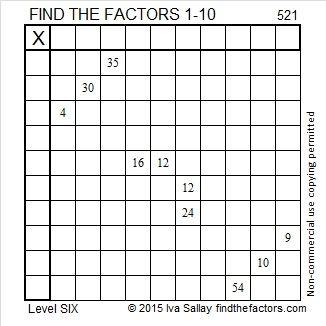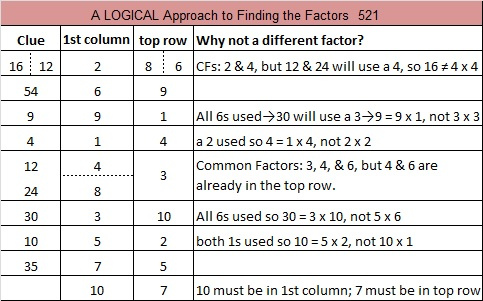# 521 and Level 6

521 = 20² + 11², and 521  is the hypotenuse of the primitive Pythagorean triple 279-440-521.Print the puzzles or type the solution on this excel file: 10 Factors 2015-06-08

—————————————————————————————————

• 521 is a prime number.
• Prime factorization: 521 is prime.
• The exponent of prime number 521 is 1. Adding 1 to that exponent we get (1 + 1) = 2. Therefore 521 has exactly 2 factors.
• Factors of 521: 1, 521
• Factor pairs: 521 = 1 x 521
• 521 has no square factors that allow its square root to be simplified. √521 ≈ 22.8254244How do we know that 521 is a prime number? If 521 were not a prime number, then it would be divisible by at least one prime number less than or equal to √521 ≈ 22.8. Since 521 cannot be divided evenly by 2, 3, 5, 7, 11, 13, 17, or 19, we know that 521 is a prime number.

—————————————————————————————————